Art of Problem Solving textbooks have been used by outstanding students since 1993. This set of books is written by Richard Rusczyk and Sandor Lehoczky.

In the set of Art of Problem Solving textbooks, math problems are ranging from introductory to Olympiad level to challenge all types of middle school students looking to learn from their books.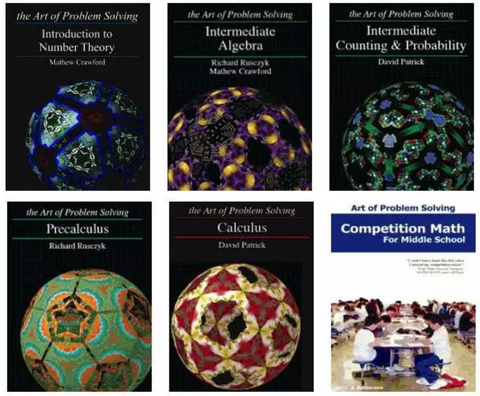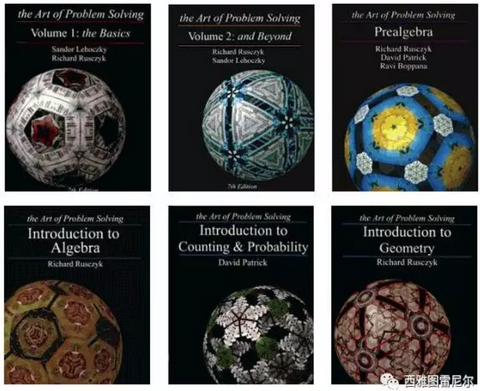The contents of AoPS textbooks are very comprehensive: algebra, geometry, statistics, probability, number theory, calculus, etc.. This set of textbooks is divided into three categories according to the degree: entry, advanced improvement, and competition preparation. Each piece of the catalogue is a textbook, and each textbook is accompanied by an answer book (Solutions Manual).

Below is the "map" of all AoPS textbooks. The top row of the map consists of the core contents, which parallels the standard prealgebra-to-calculus school curriculum, but in much greater depth both in mathematical content and in problem-solving skills. We recommend that students proceed through AoPS core curriculum in left-to-right order, supplementing with non-core books as desired.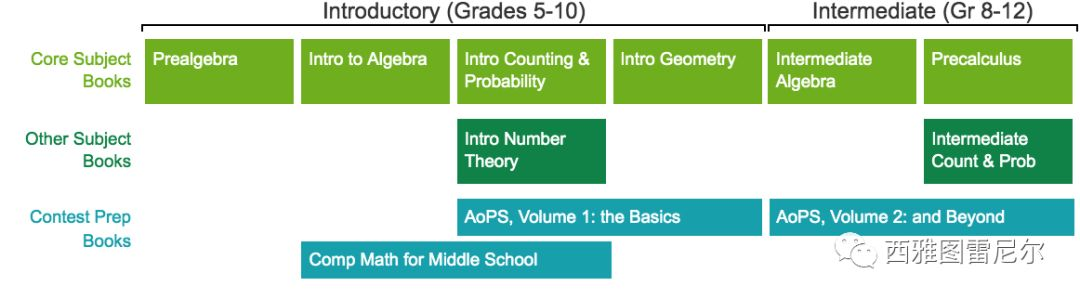Each textbook starts with concepts and principles of math. After clarifying a concept, it is accompanied by some examples and math problems.

AoPS textbooks clarifying a concept is so clear that students do not need to look for assistance if they read books carefully enough.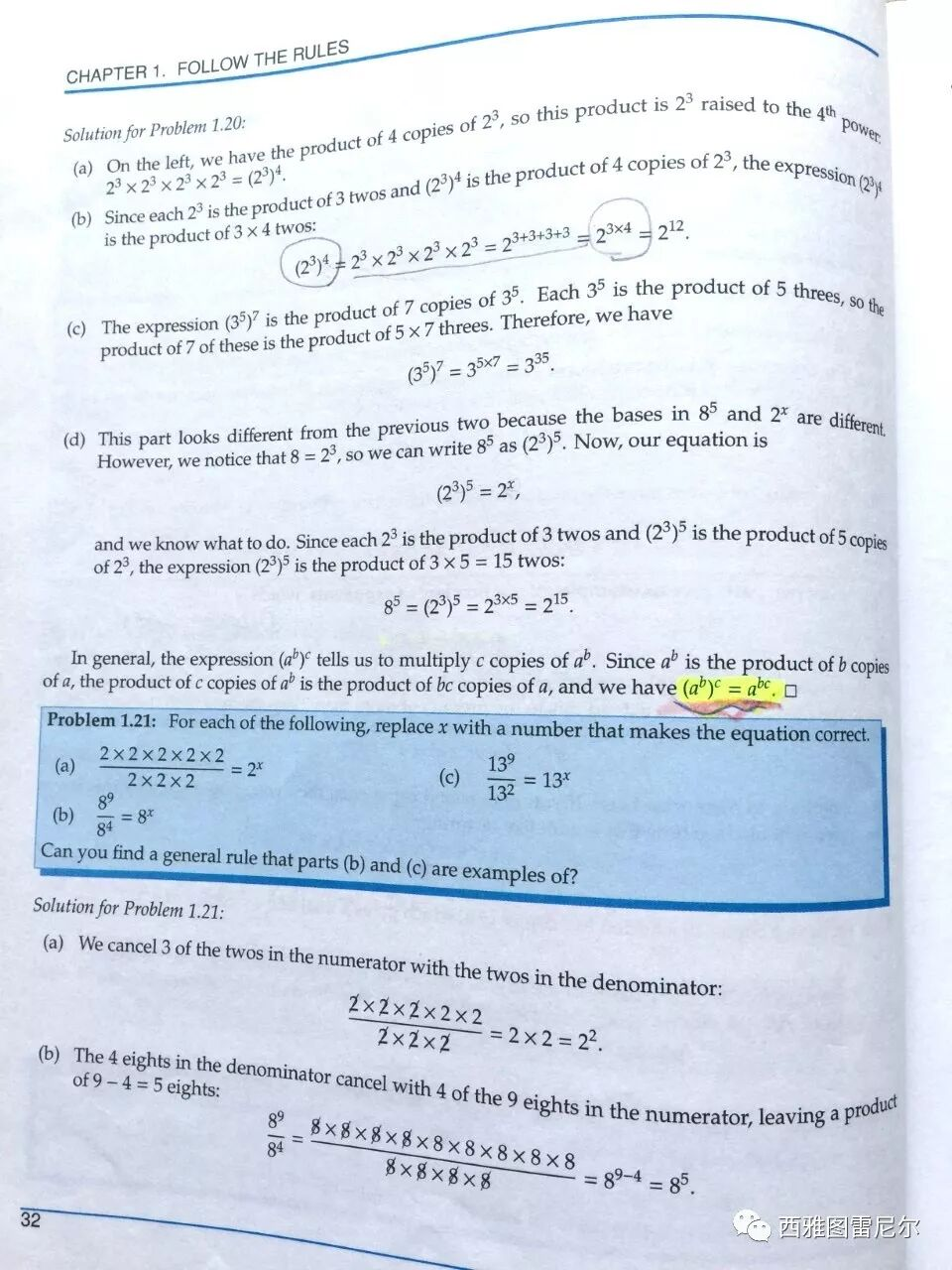The explanations for the examples are very detailed as well. They never miss any problem-solving step, but let the students clearly see how each step is obtained.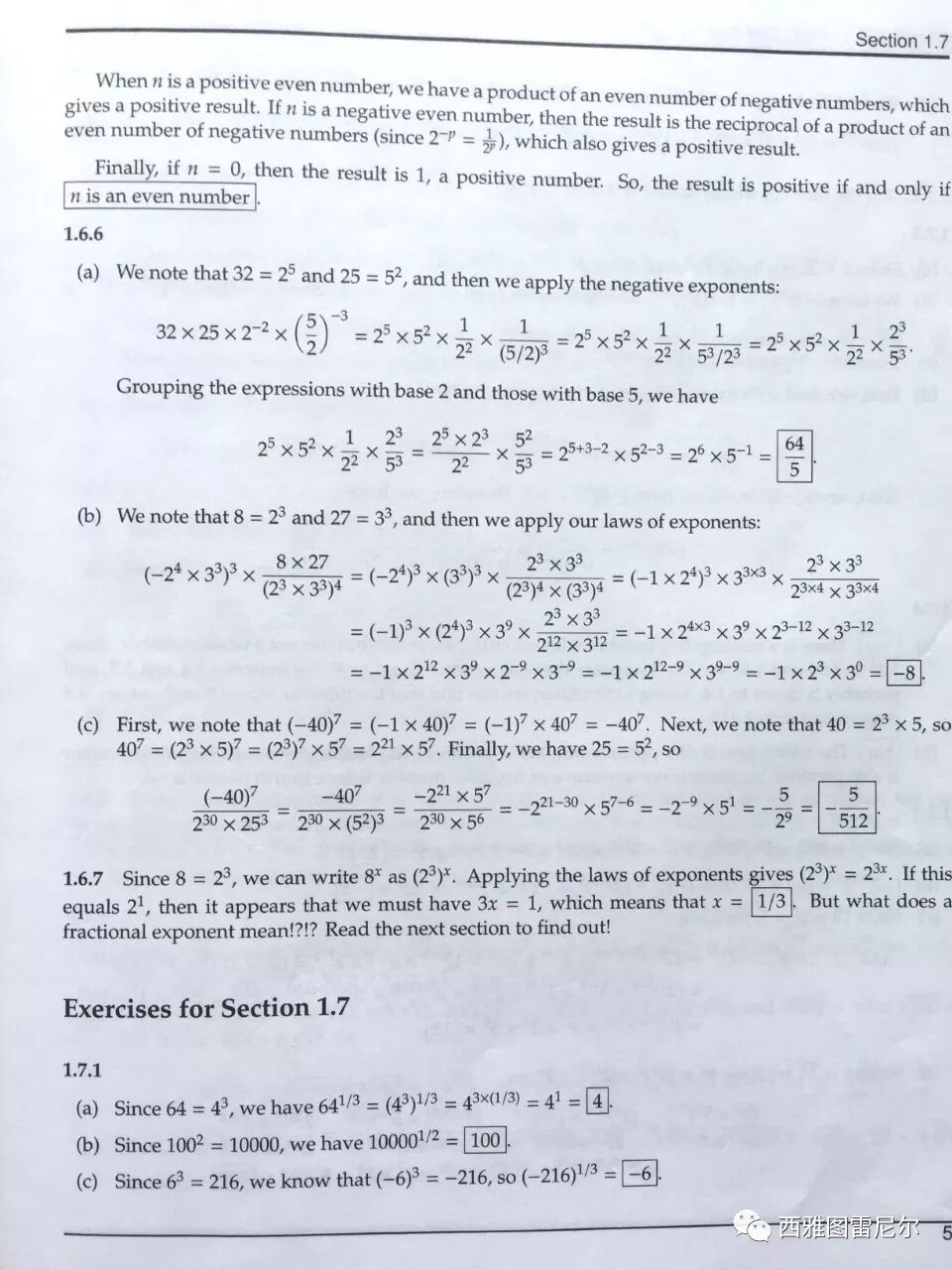If there is a special need to remind students, "Warning" and "Important" will be marked.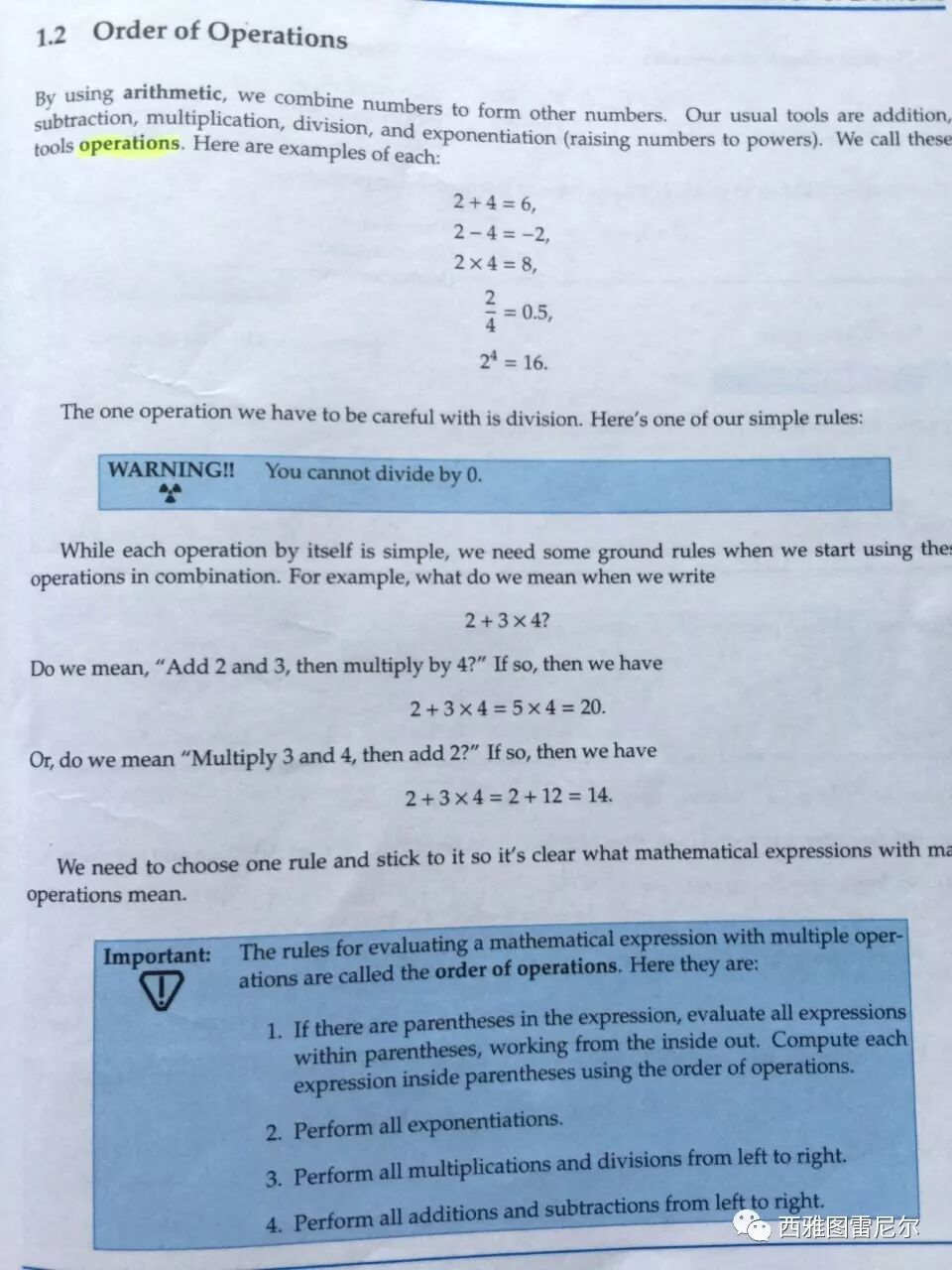A few math problems are listed for students to solve. The problems are from easy to hard, they present a much broader and deeper exploration of challenging mathematics.The easy ones are basic math problems. The difficult problems have reached the standards of MATHCOUNTS and AMC in the middle school mathematics competition. The answers to the problems are in the matched solutions manuals.The set of Art of Problem Solving books is worth exploring for yourself. It is definitely a treasure trove of mathematics.